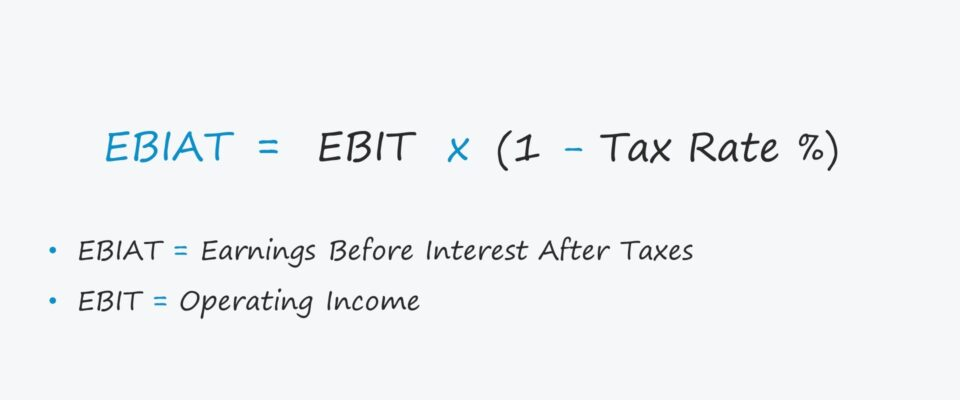Welcome to Wall Street Prep! Use code at checkout for 15% off.# EBIAT

Guide to Understanding EBIAT## How to Calculate EBIAT?

EBIAT, short for “Earnings Before Interest After Taxes”, represents a company’s profit if no debt-related tax benefits were received.

Since the impact of financing differences in capital structures is removed, comparisons among different companies is more “apples to apples”.

In practice, the EBIAT metric – also referred to as net operating profit after taxes (NOPAT) – is used to estimate a company’s operating profits once the effects of financing items, namely interest expense, are removed.

If the impact of debt is not removed, the discretionary decisions surrounding the amount of leverage among the peer set could skew the calculations, resulting in misleading findings.

Interest expense is tax-deductible, so the company of taxes paid are reduced by the so-called “interest tax shield”.

Calculating EBIAT is one of the first steps in projecting a company’s future free cash flows (FCFs) in a DCF model because it is an unlevered metric.

The metric should reflect a company’s taxed core operating income (EBIT), after eliminating the impact of non-operating gains / (losses) and debt financing (e.g. “tax shield”), i.e. normalized under the assumption that the company’s capitalization is entirely all-equity with no debt.

## EBIAT Formula

EBIAT represents the profits available to all sources of capital, i.e. both debt and equity.

• Debt – Banks, Financial Institutions, Direct Lenders
• Equity – Common Shareholders, Preferred Stockholders

The formula multiplies operating income (EBIT) by (1 – t), in which “t” is the company’s marginal tax rate.

EBIT is a company’s gross profit minus all operating expenses, with includes items such as depreciation, amortization, employee compensation, and overhead costs.

Moreover, while the marginal tax rate is used here, the effective tax rate (i.e. the actual tax rate paid based on historical periods), could also be used.

EBIAT = EBIT x (1Tax Rate %)

An alternative formula starts with net income, as shown below.

EBIAT = (Net Income + Non-Operating Losses Non-Operating Gains + Interest Expense + Taxes) x (1 Tax Rate %)
• Step 1. Beginning with net income, we first add back non-operating losses and subtract non-operating gains.
• Step 2. Next, we add back the impact of interest expense (i.e. the cost of debt financing) and taxes.
• Step 3. Upon doing so, we have gone from net income up to the operating income (EBIT) line item, i.e. just as in the first formula.
• Step 4. The net income metric is affected by non-core income / (losses), interest expense, and taxes – hence, we went through the process of removing the impact of those line items.
• Step 5. The final step is to then multiply EBIT by (1 – tax rate).

## EBIAT Calculation Example

Suppose we have two companies that share the following financials:

Down to the operating income (EBIT) line, the two companies are identical.

• Gross Profit = \$140 million
• Operating Income (EBIT) = \$100 million

But the similarities end there because of a non-operating line item, interest expense.

Here, we’ll assume the two companies carry different amounts of debt on their balance sheet.

• Company A (All-Equity Firm) = \$0 Interest Expense
• Company B (Equity-Debt Firm) = \$50 million of Interest Expense

The interest tax shield subsequently reduces Company B’s pre-tax income.

• Company A Pre-Tax Income = \$100 million
• Company B Pre-Tax Income = \$50 million

The \$50 million difference is caused by the interest expense, and the taxes of the two companies vary because of the tax deductibility of interest.

Given a 20% tax rate assumption, the companies pay the following taxes:

• Company A Taxes Paid = \$20 million
• Company B Taxes Paid = \$10 million

In conclusion, the taxes paid by Company A are double that of Company B, and the net incomes of the two companies are shown below.

• Company A Net Income = \$80 million
• Company B Net Income = \$40 millionStep-by-Step Online Course

### Everything You Need To Master Financial Modeling

Enroll in The Premium Package: Learn Financial Statement Modeling, DCF, M&A, LBO and Comps. The same training program used at top investment banks.